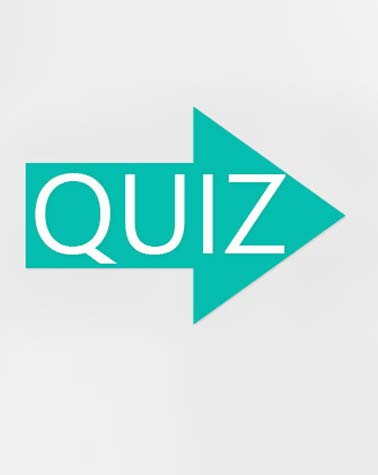# Speed, Distance, Time Quiz

10 Questions | Total Attempts: 338Settings.

• 1.
What signifies "how far" something has traveled?
• A.

Speed

• B.

Distance

• C.

Time

• D.

None

• 2.
________ signifies "how fast" an object is going.
• A.

Distance

• B.

Time

• C.

Speed

• D.

None

• 3.
A car travels 12 miles per hour for 10 hours.  How far did it travel?
• A.

1.2 miles

• B.

120 miles

• C.

0.83 miles

• D.

100 miles

• 4.
The unit for speed is miles, true or false?
• A.

True

• B.

False

• 5.
Mike flies 400 kilometers in 5 hours.  How fast was he going?
• A.

125 km per hour

• B.

80 km per hour

• C.

2000 km per hour

• D.

16 km per hour

• 6.
Joshua jogs for 2 hours at a rate of 12 mph.  What are you trying to find?
• A.

Speed

• B.

Time

• C.

Distance

• D.

None

• 7.
In the pyramid for speed, distance and time, what 2 must be multiplied?
• A.

Speed and Time

• B.

Distance and Time

• C.

Speed and Distance

• D.

None

• 8.
If Marty ran for 4 hours yesterday and completed 96 miles, he was going [Blank] miles per hour.
• 9.
What signifies "how long" your activity took?
• A.

Distance

• B.

Speed

• C.

Rate

• D.

TimeBack to top